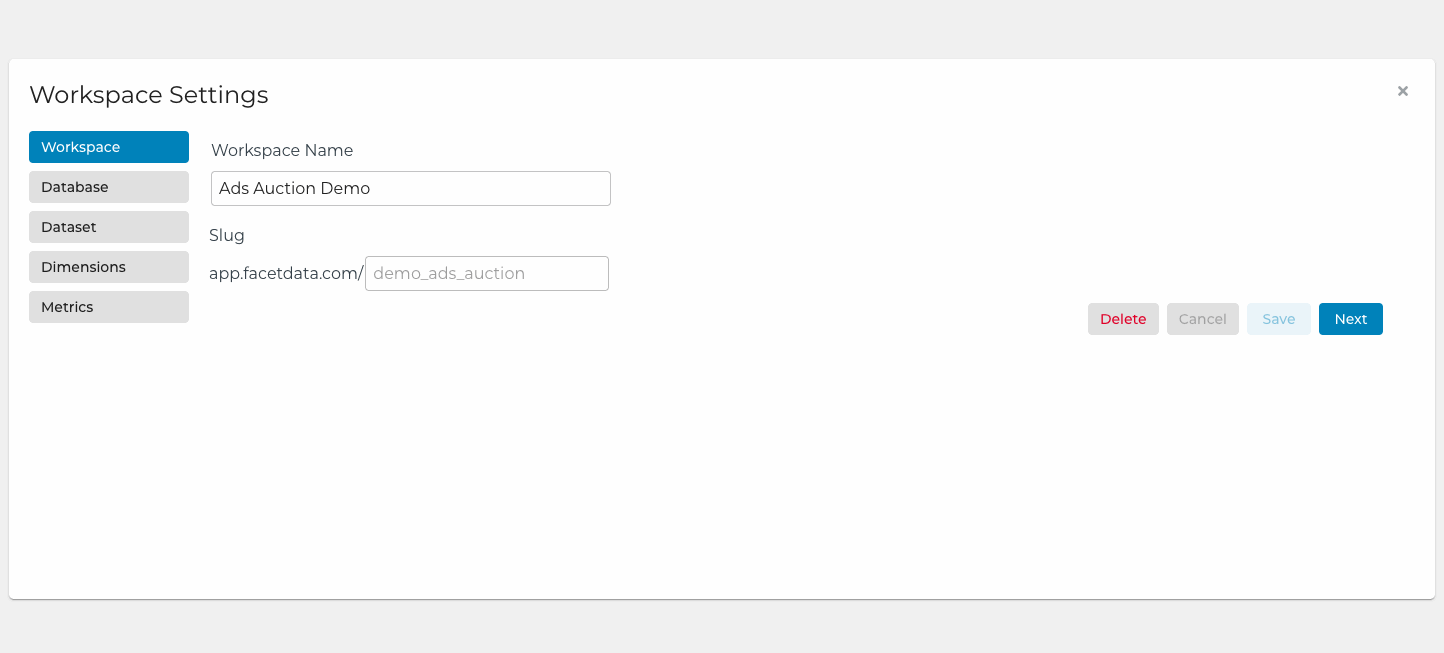# Creating custom metrics

Create custom calculated metrics for your workspace in the Metrics tab of your Workspace Settings page. Simply click "Add New Metric" to get started.The "Calculation" field supports standard arithmetic operators (addition, subtraction, division, multiplication) and basic aggregate functions.

# Available functions

In addition to the standard arithmetic operators, the following aggregation functions are available:

• `.sum( \$variable )`
• `.count()`
• `.countDistinct( \$variable )`
• `.filter( boolean_expression )`
• `.average( \$variable )`

Functions can be chained. For example, let's say you have a set of event records that includes purchase events. To count the number of distinct users who have completed purchase events using cash, you would use:

`\$main.filter(\$event == 'PURCHASE' and \$payment == 'CASH').countDistinct(\$user_id)`

# Calculation cheat sheet

Here's a cheat sheet with some common metrics we see in workspaces. Copy what works for you (making sure to replace all `\$variables` with your respective column names) or just use these as a jumping off point.

Description

Calculation

Count of all records

`\$main.count()`

Margin

`\$main.sum(\$charge_price) - \$main.sum(\$publisher_revenue)`

CTR

`\$main.sum(\$clicks) / \$main.sum(\$impressions)`

eCPM

`1000 * \$main.sum(\$ad_revenue) / \$main.sum(\$impressions)`

Unique users

`\$main.countDistinct(\$user_id)`

Unique visitors

`\$main.countDistinct(\$anonymous_id)`

OK Response rate

`\$main.filter(\$response_http_status==200).count() / \$main.count()`

Is open auction rate

`\$main.filter(\$bid_dealid.is(null)).count() / \$main.count()`